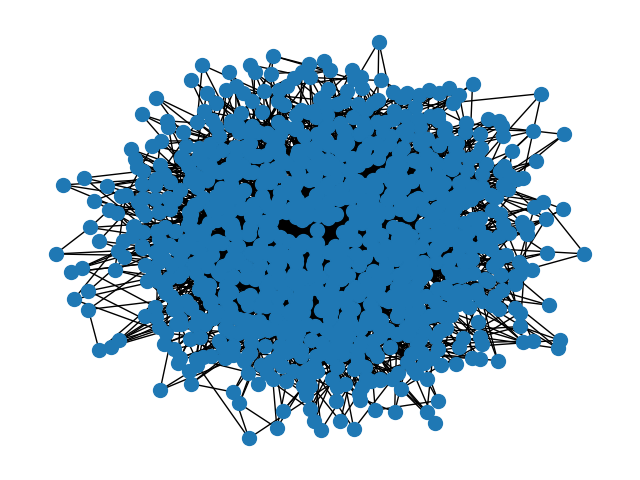# Parallel Betweenness¶

Example of parallel implementation of betweenness centrality using the multiprocessing module from Python Standard Library.

The function betweenness centrality accepts a bunch of nodes and computes the contribution of those nodes to the betweenness centrality of the whole network. Here we divide the network in chunks of nodes and we compute their contribution to the betweenness centrality of the whole network.

Note: The example output below shows that the non-parallel implementation is faster. This is a limitation of our CI/CD pipeline running on a single core.

Depending on your setup, you will likely observe a speedup.Out:

```Computing betweenness centrality for:
Graph with 1000 nodes and 2991 edges
Parallel version
Time: 2.0864 seconds
Betweenness centrality for node 0: 0.13048
Non-Parallel version
Time: 3.5418 seconds
Betweenness centrality for node 0: 0.13048

Computing betweenness centrality for:
Graph with 1000 nodes and 4962 edges
Parallel version
Time: 2.6508 seconds
Betweenness centrality for node 0: 0.00179
Non-Parallel version
Time: 4.5647 seconds
Betweenness centrality for node 0: 0.00179

Computing betweenness centrality for:
Graph with 1000 nodes and 2000 edges
Parallel version
Time: 1.8492 seconds
Betweenness centrality for node 0: 0.00312
Non-Parallel version
Time: 3.1662 seconds
Betweenness centrality for node 0: 0.00312
```

```from multiprocessing import Pool
import time
import itertools

import matplotlib.pyplot as plt
import networkx as nx

def chunks(l, n):
"""Divide a list of nodes `l` in `n` chunks"""
l_c = iter(l)
while 1:
x = tuple(itertools.islice(l_c, n))
if not x:
return
yield x

def betweenness_centrality_parallel(G, processes=None):
"""Parallel betweenness centrality  function"""
p = Pool(processes=processes)
node_divisor = len(p._pool) * 4
node_chunks = list(chunks(G.nodes(), int(G.order() / node_divisor)))
num_chunks = len(node_chunks)
bt_sc = p.starmap(
nx.betweenness_centrality_subset,
zip(
[G] * num_chunks,
node_chunks,
[list(G)] * num_chunks,
[True] * num_chunks,
[None] * num_chunks,
),
)

# Reduce the partial solutions
bt_c = bt_sc
for bt in bt_sc[1:]:
for n in bt:
bt_c[n] += bt[n]
return bt_c

G_ba = nx.barabasi_albert_graph(1000, 3)
G_er = nx.gnp_random_graph(1000, 0.01)
G_ws = nx.connected_watts_strogatz_graph(1000, 4, 0.1)
for G in [G_ba, G_er, G_ws]:
print("")
print("Computing betweenness centrality for:")
print(nx.info(G))
print("\tParallel version")
start = time.time()
bt = betweenness_centrality_parallel(G)
print(f"\t\tTime: {(time.time() - start):.4F} seconds")
print(f"\t\tBetweenness centrality for node 0: {bt:.5f}")
print("\tNon-Parallel version")
start = time.time()
bt = nx.betweenness_centrality(G)
print(f"\t\tTime: {(time.time() - start):.4F} seconds")
print(f"\t\tBetweenness centrality for node 0: {bt:.5f}")
print("")

nx.draw(G_ba, node_size=100)
plt.show()
```

Total running time of the script: ( 0 minutes 23.652 seconds)

Gallery generated by Sphinx-Gallery